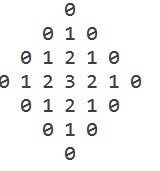# C Program for diamond pattern with different layers

CServer Side ProgrammingProgramming

Given with the number and the task is to generate the diamond pattern with the given n different layers and display it.

## Example

Input: n = 3

Output:Approach used in the below program is as follows

• Input the number of rows
• And in this pattern there are ((2 * n) + 1) rows
• Number of spaces from 0 – n is (2 * (n – i))
• And the number of spaces from n+1 till end are ((i – n) * 2)

## Algorithm

Start
Step 1-> declare a function to print a pattern
void print_pattern(int n)
declare variables as int i, j
Loop For i = 1 i <= n * 2 i++
Print space
End
Print \n
Loop For i = 1 and i <= (n * 2) – 1 and i++
IF i<n
Loop For j = 1 and j <= (n - i) * 2 and j++
Print space
End
End
Else
Loop For j = 1 and j <= (i % n) * 2 and j++
Print space
End
IF i < n
Loop For j = 0 and j <= i % n and j++
Print j
End
Loop For j = (i % n)-1 and j > 0 and j--
Print j
End
Print 0
End
Else IF i > n
Loop For j = 0 and j <= n – (i – n) and j++
Print j
End
Loop For j = (n – (i – n))-1 and j > 0 and j--
Print j
End
Print 0
End
Else
Loop For j = 0 and j <= n and j++
Print j
End
Loop For j = n –1 and j > 0 and j--
Print j
End
Print 0
End
Print \n
End
Loop For i=1 and i<=n*2 and i++
Print space
End
Print 0
Step 2-> In main()
Declare variable as int n=3
Call function print_pattern(n)

## Example

Live Demo

#include <stdio.h>
void print_pattern(int n) {
// putting the space in line 1
int i, j;
for ( i = 1; i <= n * 2; i++)
printf(" ");
printf("0\n");
// generating the middle pattern.
for ( i = 1; i <= (n * 2) - 1; i++) {
// printing the increasing pattern
if (i < n) {
for ( j = 1; j <= (n - i) * 2; j++)
printf(" ");
} else {
for ( j = 1; j <= (i % n) * 2; j++)
printf(" ");
}
if (i < n) {
for ( j = 0; j <= i % n; j++)
printf("%d ", j);
for ( j = (i % n) - 1; j > 0; j--)
printf("%d ", j);
printf("0");
}
// printing the decreasing pattern
else if (i > n) {
for ( j = 0; j <= n - (i - n); j++)
printf("%d ", j);
for ( j = (n - (i - n)) - 1; j > 0; j--)
printf("%d ", j);
printf("0");
} else {
for ( j = 0; j <= n; j++)
printf("%d ", j);
for ( j = n - 1; j > 0; j--)
printf("%d ", j);
printf("0");
}
printf("\n");
}
// putting the space in last line
for ( i = 1; i <= n * 2; i++)
printf(" ");
printf("0");
}
int main() {
int n = 3;
print_pattern(n);
return 0;
}

## Output